# Wave Equation

(redirected from The wave equation)
Also found in: Dictionary, Thesaurus.
Related to The wave equation: diffraction, Solving the wave equation, Standing waves

## Wave equation

The name given to certain partial differential equations in classical and quantum physics which relate the spatial and time dependence of physical functions. In this article the classical and quantum wave equations are discussed separately, with the classical equations first for historical reasons.

In classical physics the name wave equation is given to the linear, homogeneous partial differential equations which have the form of Eq. (1).

(1)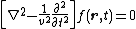Here &ugr; is a parameter with the dimensions of velocity; r represents the space coordinates x, y, z; t is the time; and ∇2 is Laplace's operator defined by
(2)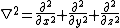Eq. (2). The function f( r ,t) is a physical observable; that is, it can be measured and consequently must be a real function.

The simplest example of a wave equation in classical physics is that governing the transverse motion of a string under tension and constrained to move in a plane.

A second type of classical physical situation in which the wave equation (1) supplies a mathematical description of the physical reality is the propagation of pressure waves in a fluid medium. Such waves are called acoustical waves, the propagation of sound being an example. A third example of a classical physical situation in which Eq. (1) gives a description of the phenomena is afforded by electromagnetic waves. In a region of space in which the charge and current densities are zero, Maxwell's equations for the photon lead to the wave equations (3).

(3)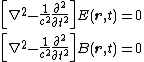Here E is the electric field strength and B is the magnetic flux density; they are both vectors in ordinary space. The parameter c is the speed of light in vacuum. See Electromagnetic radiation, Maxwell's equations

The nonrelativistic Schrödinger equation is an example of a quantum wave equation. Relativistic quantum-mechanical wave equations include the Schrödinger-Klein-Gordon equation and the Dirac equation. See Quantum mechanics, Relativistic quantum theory

McGraw-Hill Concise Encyclopedia of Physics. © 2002 by The McGraw-Hill Companies, Inc.
The following article is from The Great Soviet Encyclopedia (1979). It might be outdated or ideologically biased.

## Wave Equation

a partial differential equation that describes the process of propagation of a disturbance in a medium. In the case of small disturbances and a homogeneous, isotropic medium, the wave equation has the form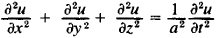where x, y, and z are spatial variables; t is time; u = u(x, y, z) is the function to be determined, which characterizes the disturbance at point (x, y, z) and time t; and a is the velocity of propagation of the disturbance. The wave equation is one of the fundamental equations of mathematical physics and is applied extensively. If u is a function of only two (one) spatial variables, then the wave equation is simplified and is called a two-dimensional (one-dimensional) equation. It permits a solution in the form of a“diverging spherical wave”:

u = f(t – r/a)/r

where f is an arbitrary function and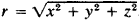. The so-called elementary solution (elementary wave) is of particular interest:

u = δ(t - r/a)/r

(where δ is the delta function); it gives the process of propagation for a disturbance produced by an instantaneous point source acting at the origin (when t = 0). Figuratively speaking, an elementary wave is an“infinite surge” on a circumference r = at that is moving away from the origin at a velocity a with gradually diminishing intensity. By superimposing elementary waves it is possible to describe the process of propagation of an arbitrary disturbance.

Small vibrations of a string are described by a one-dimensional wave equation: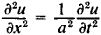In 1747, J. d’Alembert proposed a method of solving this wave equation in terms of superimposed forward and back waves: u = f(x - at) + g(x + at); and in 1748, L. Euler established that the functions f and g are determined by as-signing so-called initial conditions.

### REFERENCE

Tikhonov, A. N., and A. A. Samarskii. Uravneniia matematicheskoi fiziki, 3rd ed. Moscow, 1966.

P. I. LIZORKIN

## wave equation

[′wāv i‚kwā·zhən]
(physics)
In classical physics, a special equation governing waves that suffer no dissipative attenuation; it states that the second partial derivative with respect to time of the function characterizing the wave is equal to the square of the wave velocity times the Laplacian of this function. Also known as classical wave equation; d'Alembert's wave equation.
Any of several equations which relate the spatial and time dependence of a function characterizing some physical entity which can propagate as a wave, including quantum-wave equations for particles.
McGraw-Hill Dictionary of Scientific & Technical Terms, 6E, Copyright © 2003 by The McGraw-Hill Companies, Inc.
References in periodicals archive ?
This consists in replacing time-derivatives through multiplication by the term i[omega] (see, e.g., , Section 1.2), and solve the wave equation by looking for eigenvalues of the Laplace operator.
In this paper, applying ideas from [16,18], we investigate the generalized Hyers-Ulam stability of the wave equation (3) with a source, where x > a and t > b with a,b [member of] R [union] {-[infinity]}.
Separating the variables of the wave equation and applying the well known Damour-Ruffini-Sannan method, we determine accurately the thermal radiation and chemical potential at the event horizon r = h in general nonstationary black hole.
If, however, the microstructure has more complicated mechanical properties, then it might be physically expedient to start with stress-strain relations and to use the Cauchy method for deriving the wave equations. For example, in case of felt materials the behaviour of the material is hereditary [56,57].
The wave equation formulations also permit locally varying field order, locally varying constitutive parameters, and are compatible with exact radiating boundary conditions (ERBCs).
However, there is an important lack of research on absorbing boundary conditions for the wave equation. In fact, the most popular absorbing boundary condition, the so-called perfectly matched layer, has been always developed to be used on Euler equations, but very few attempts to use them on the wave equation can be found in the PSTD technical literature.
For illustrating the concepts we adopt one example for solving the wave equation on Cantor sets with local fractional operator.
In three-dimensional setting in coordinates x, y, z the wave equation is
But the positron cannot survive in the Cosmos because its function is multiplied by [A.sub.0] = 0 into the fundamental eigenvector of the Wave Equation: [R.sub.0](r)= 1/r{[sin(kr)] = [A.sub.0]) [cos(kr)]} obtained through the boundary conditions R0(re) = 0 and [R.sub.0] (r[right arrow][infinity]) = 0 (being [r.sub.e] = the radius of the electron).
H., An Explicit difference method for the wave equation with extended stability range, BIT, 19, 378-383(1979).
The wave equation remains invariant under the transform t [right arrow] -t in a lossless medium.
It is well known that one of the purposes of integral transforms like Fourier, Laplace and Mellin is to solve differential equations like the Heat equation the wave equation and some EDDs.

Site: Follow: Share:
Open / Close